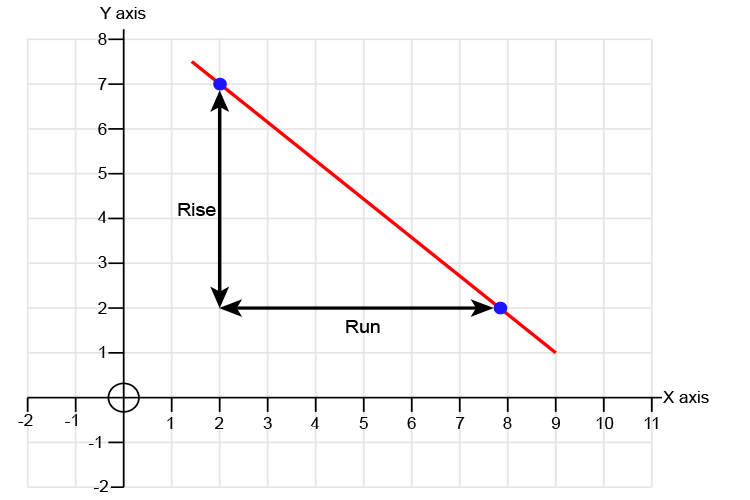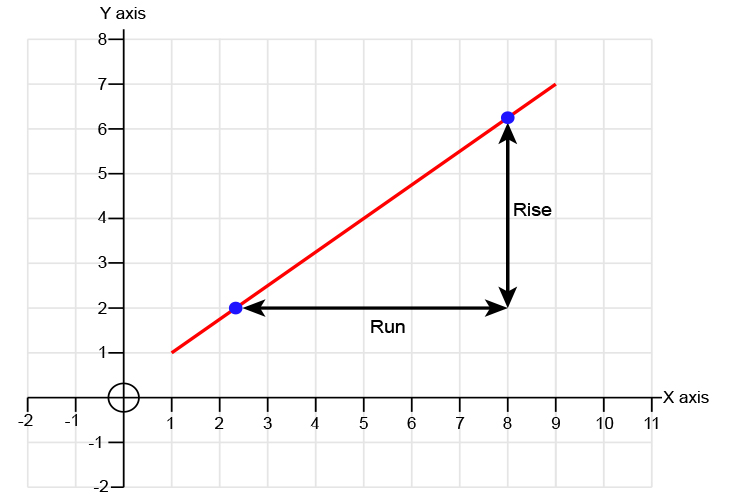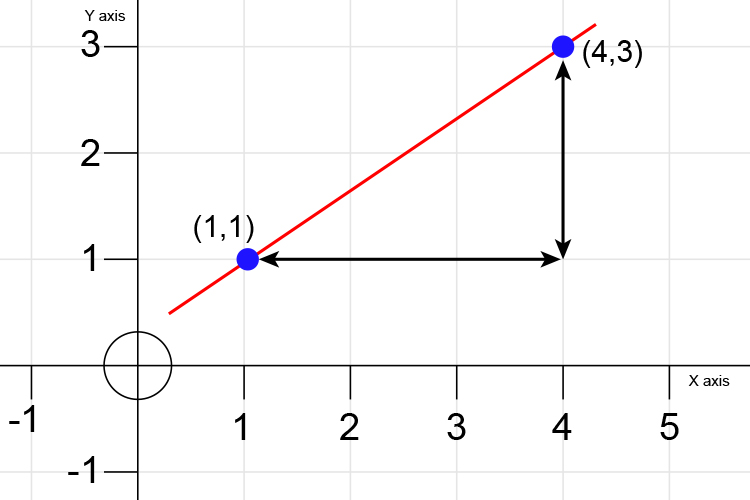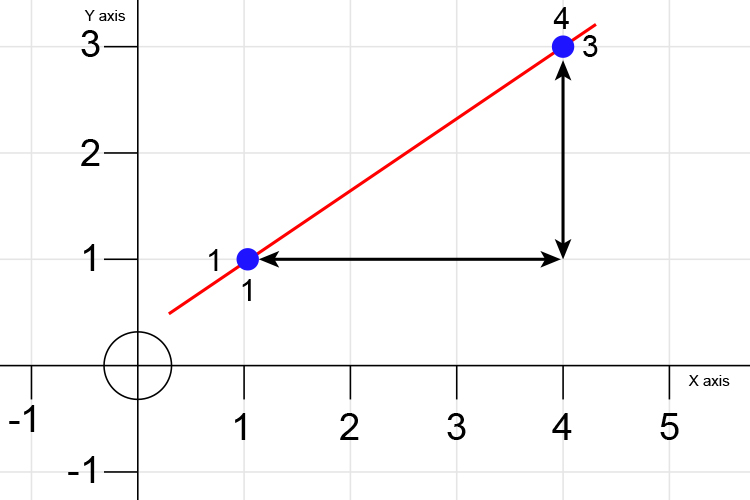# Gradient – Rise over run

To calculate the gradient of any line select any 2 points on the line and find the rise and run.

Because:

Gradient=(Rise)/(Run)    (Gradient is Rise over Run)It's grey (gradient) and then the sun rises overhead (rise over) as we go for a run (run).

A graph showing a slopeA graph showing an alternative slopeYou can run in either direction.

Example

Find the gradient of the graph below:Rise=3-1=2

Run=4-1=3

Therefore Gradient=(Rise)/(Run)

Gradient=2/3=0.66